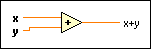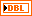# Add Function

## LabVIEW 2018 Help

Edition Date: March 2018
Part Number: 371361R-01
View Product Info

### DOWNLOAD (Windows Only)LabVIEW 2016 HelpLabVIEW 2017 HelpLabVIEW 2018 HelpLabVIEW 2019 HelpLabVIEW 2020 Help

Owning Palette: Numeric Functions

Requires: Base Development System

Computes the sum of the inputs.

If you wire two waveform values or two dynamic data type values to this function, error in and error out terminals appear on the function. You cannot add two time stamp values together. The dimensions of two matrices you want to add must be the same. Otherwise, this function returns an empty matrix. The connector pane displays the default data types for this polymorphic function.x can be a scalar number, array or cluster of numbers, array of clusters of numbers, and so on.y can be a scalar number, a fixed-point number, an array or cluster of numbers, an array of clusters of numbers, a time stamp, and so on.x+y is the sum of x and y.Note  You can manually configure this function to output data of a type you want. To specify the output data type, right-click the function and select Properties to display the Object Properties dialog box. On the Output Configuration page, click the Representation icon and select the data type you want. A blue coercion dot appears on the output terminal of the function to indicate that you have configured the output data type.

## Add Details

When you wire matrix data as an input to this function, a VI that includes subVIs that work with the matrix data type replaces the function. The resulting VI has the same icon but contains a matrix-specific algorithm. The node remains a VI if you disconnect the matrix from the input(s). Wire other data types as inputs to restore the original function. If you wire a data type to a function and that data type causes a basic math operation to fail, the function returns an empty matrix or NaN.

You also can add two or more values using the Compound Arithmetic function.

The Numeric Functions VI at the bottom of the page shows how the Add function operates with different data types.

### Fixed-Point Details

If you wire fixed-point values to Numeric functions such as Add, Subtract, Multiply, and Square, the functions usually return values that do not lose any bits of word length. However, if the operation creates a value that exceeds the maximum word length that LabVIEW accepts, overflow or rounding conditions can occur. LabVIEW accepts a maximum word length of 64 bits. Use the Numeric Node Properties dialog box to configure how LabVIEW handles overflow or rounding of fixed-point data.

## Example

Refer to the Numeric Functions VI in the labview\examples\Numerics directory for an example of using the Add function.

Not Helpful